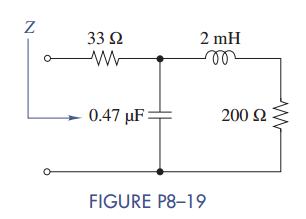Chat Now

# Find the equivalent impedance Z in Figure P8–19 when ω = 50 krad/s. What two elements (R, L,...

### Find the equivalent impedance Z in Figure P8–19 when ω = 50 krad/s. What two elements (R, L,...

Find the equivalent impedance Z in Figure P8–19 when ω = 50 krad/s. What two elements (R, L, and/or C) could be used to replace the phasor circuit?manish jayant 27-Jul-2020Get solution